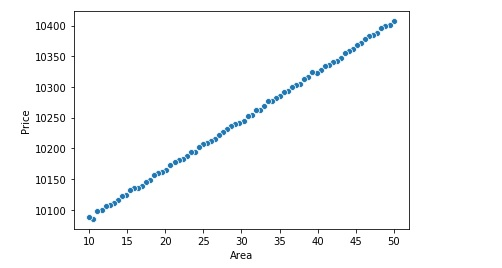# Linear Regression using sklearn in 10 lines

Linear regression is one of the most popular and fundamental machine learning algorithm. If relationship between two variables are linear we can use Linear regression to predict one variable given that other is known.

For example if we are researching how the price of the house will vary if we change the area of the house we can use Linear Regression. Here area of the house variable may be denoted by x ( independent variable) and price of the house may denoted by variable y ( dependent variable).

There are many use cases of Linear Regression model like estimating the house price based upon the area, sales forecasting based on the advertising investment etc.

Let us implement a simple linear regression in python where we have one feature as house area and the target variable is housing price.

You may like to watch a video on Linear Regression from Scratch in Python

Import the libraries

First we need to import the required libraries as below

``````import numpy as np
import pandas as pd
import seaborn as sns
from sklearn.linear_model import LinearRegression
from sklearn.metrics import r2_score,mean_squared_error``````

Then let us load the data in jupyter notebook using below code

``````df = pd.read_csv('Linear-Regression-Data.csv')

Define and Train the Linear Regression Model

``````x = df.x.values.reshape(-1, 1)
y = df.y.values.reshape(-1, 1)
x_train, x_test, y_train, y_test = train_test_split(x, y, test_size=0.30, random_state=42)

linear_model = LinearRegression()
linear_model.fit(x_train,y_train)``````

Predict the Values using Linear Model

``y_pred = linear_model.predict(x_test)``

Evaluate the Model

For evaluating the Linear Regression Model, we generally calculate two main metrics namely R-Squared and RMSE (Root Mean Squared Error).

``````r2_score(y_test, y_pred)
mse = mean_squared_error(y_test, y_pred)
rmse = np.sqrt(mse)``````